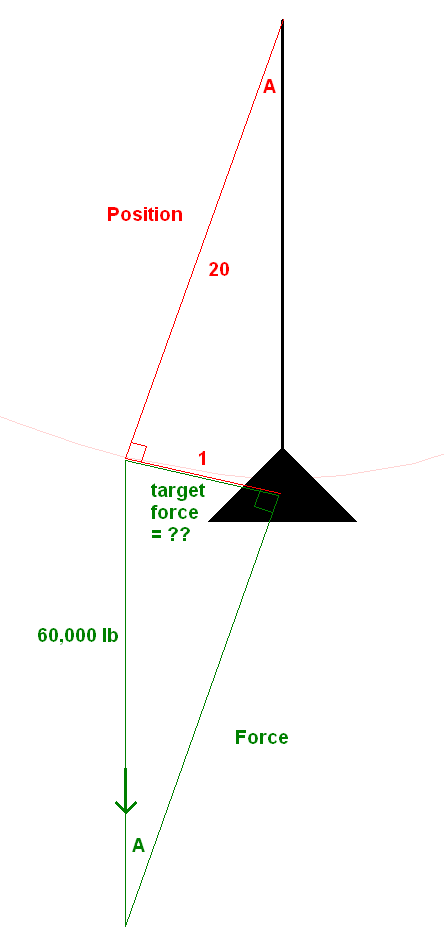User Name Remember Me? Password

 General Physics General Physics Help ForumSep 15th 2019, 06:45 PM #1 Junior Member   Join Date: Sep 2019 Posts: 1 Need help. Need to figure out the force required to move this vessel about a foot , I understand limited info . But just need ball park Force required to pull it . Please show work so I can understand it , it’s hung by chain falls and hanging off groundSep 16th 2019, 03:56 AM #2 Senior Member   Join Date: Jun 2016 Location: England Posts: 1,060 Force = Mass x Acceleration Note that this implies that it is not a question of; How much Force is needed to move the Mass, it is how much Force is required to move the Mass in a sensible Time? It is a bit awkward that you have given the weight in pounds Is that pounds mass or founds force? topsquark likes this. __________________ ~\o/~Oct 4th 2019, 08:23 PM #3 Junior Member   Join Date: Sep 2019 Location: low nuclear orbit Posts: 20 Well technically almost zero. Because F=MA, and you didn't specify just how quickly you'd have to move it by 1 foot. If it can take all day, then you would need almost no force at all, because as long as you get even a LITTLE acceleration, the weight will eventually move that 1 foot. However, since it's on a pendulum, the more you move it horizontally, it also goes slightly higher vertically, so the weight's gravity will want to keep pulling the weight back to the middle where it started, so "0.00000001" won't actually cut it. Basically, you have to figure out the force, in Newtons or pounds or whatever, of the desire of the weight to go back to the middle, when the weight is almost 1 foot from the middle, because that's when it's at the steepest angle, and hence that's when the gravity is fighting against you the most. Gravity is downward, but you can view it has having two components: one in the direction of the slope (see https://www.physicsclassroom.com/Cla...ors/u3l3e3.gif which is for an inclined plane) and one perpendicularly into the slope. The one in the direction of the slope is the one we're looking for, because that's the force of its "desire to go back to the middle", which we need just SLIGHTLY more force than, to make it go back against that force. First we need to find the angle A. If the chain is 20 feet, and we get it 1 foot to the side, the angle's tangent (opposite / adjacent) is 1/20, so atan 1/20 = 2.86240522486162 degrees. Then the gravity's components must have that same angle A. If we want the target force, and you can see in the diagram that it's opposite side of the angle A in the lower triangle, while the 60,000 lb is the hypotenuse, then we want to multiply the 60,000 by the sine of 2.86240522486162 degrees (because sine is opposite / hypotenuse), which is 0.04993761692. So 60,000 x 0.04993761692 = 2,996 lb of gravity component force along the angle back toward the middle of the pendulum when the weight is at the 1-foot-from-the-middle mark. So we'd need 2,996 lb of force to match it. I think.Last edited by muon; Oct 4th 2019 at 08:53 PM. Reason: diagramThread ToolsShow Printable VersionEmail this Page Display ModesLinear ModeSwitch to Hybrid ModeSwitch to Threaded Mode